##1. Hyperbola created by varying parallels

Consider two points B, C on two lines b, c correspondingly intersecting at point A. Draw from B, C parallel lines intersecting lines c, b at C', B' correspondingly. These parallels can be considered to meet at a point at infinity [P]. In the figure below [P] is represented by line BP with P varying on the parallel to BC from A.
In the following properties tripoles/tripolars are taken with respect to triangle ABC. Both operations are denoted by tr. Thus, tr(P) the tripolar of a poing is a line and tr(L) the tripole of a line is a point.

 Lines B'C', for various directions of the parallels, envelope an hyperbola.
 The hyperbola has b, c as asymptotic lines. The varying triangle AB'C' is an asymptotic one of the hyperbola and, as such, has area constant and equal to that of triangle ABC.
 Let B*(resp. C*) be the harmonic conjugate of B' (resp. C') with respect to AB (resp. AC). Then line B*C* is the trilinear polar, with respect to triangle ABC, of the point at infinity determined by the direction of the parallel lines.
 Lines B'C' and B*C* intersect on line BC at a point A*. Also the pair of lines C'B* and B'C* intersect on line BC at the harmonic conjugate A2 of A* with respect to B,C.
 Let Q be the intersection point of line BC' with B*C*. The length-ratio C'Q/QB = 2.
 The tripoles S=tr(LS) of the tangents LS = B'C' of the hyprebola lie on the perspectrix L = tr(A0) of the hyperbola, which is the line joining the middles {C1,B1} of sides AB, AC (the perspector A0 being the symmetric of A with respect to the middle A1 of BC).
 Line A3A, where A3 the middle of B'C' and line A1S intersect on a point A4 lying on tr([P]). Similar incidences: {A1,B1,M'} collinear, {A1,C1,M} collinear, {C1,A4,M'} collinear, {B1,A4,M} collinear, {A2,C1,P} collinear, {A2,B1,P'} collinear.
 Lines BC' and CB', joining the non-collinear with the center (of hyperbola) vertices of any two asymptotic triangles of the hyperbola are parallel.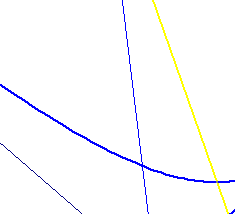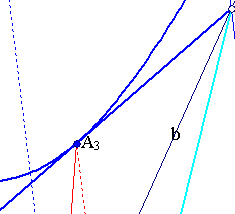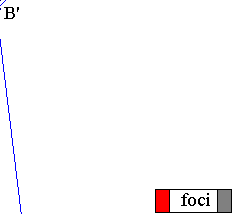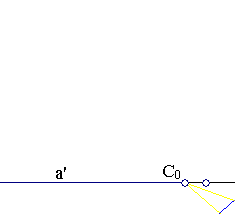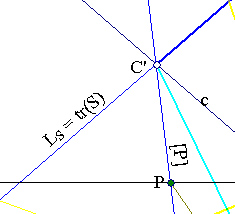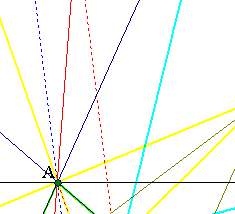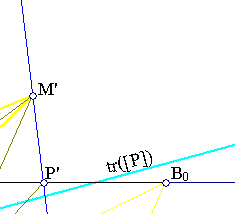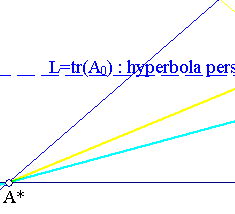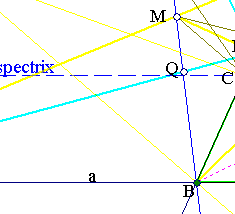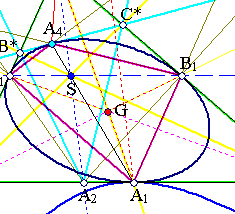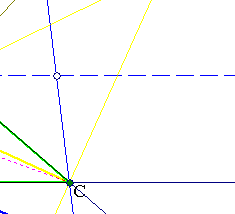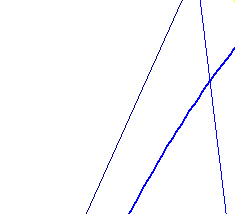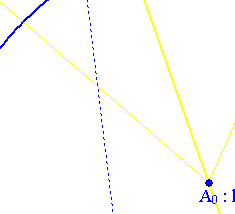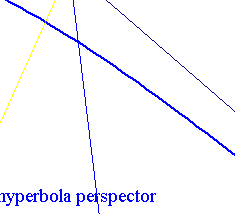The proofs are very simple. The area of the varying triangles AB'C' is equal to the area of the fixed triangle ABC (see Trapezium.html ). [1,2] follow by applying the remark made in AsymptoticTriangleInv.html .
Regarding the proof of , note that line B*C* satisfies the definition of the trilinear polar for the point at infinity (denoted in the figure by [P]) defined by the direction of parallel lines (which is the direction of BP).
Regarding , the statement on the coincidence of A* on BC follows from the fact that (B,A,B*B') and (C,A,C*,C') are two harmonic divisions on lines b, c and have A in common (see CrossRatioLines.html ). The same argument proves the coincidence of B'C* and C'B* at A2 on BC. That {A*,A2} are harmonic conjugate with respect to {B,C} follows by considering the harmonic bundle B'(C',C*,A,C).
 follows from the previous arguments and the fact that (B,M,Q,C') is a harmonic division, where M denotes the middle of BC' (the bundle of lines A*(B,M,Q,C') is a harmonic one).
To see  note first that the middles {M,M'} of {BC',CB'} are collinear with {A,A*} and build with them a harmonic division (consider the complete quadrilateral-trapezium BCB'C'). Then, {M,B*,C} are collinear as well as {B,C*,M'}. Also by definition the tripole S of B'C' is the intersection of the diagonals {MC,M'B} of trapezium BCM'M. Thus S is on line AA2, which is parallel to [P] (it is the polar of A* with respect to the pair of lines {BC',CB'}), and is also the middle of segment AA2.
To prove  note that line bundles S(B,C,A1,A*) and A(C',B',A3,A*) define the same cross ratio on every line hence on tr([P]) too. Since the two bundles define the same three points {A*,B*,C*} on this line the fourth points will coincide too. The other collinearities of  are proved analogously.  is trivial.

In the file HyperbolaPropertyParallels2.html I discuss the figure resulting from the previous one by applying to it a projectivity fixing the vertices of the triangle and mapping the centroid G to an other arbitrary point of the plane.

##2. The two inconics

 The hyperbola considered above is an inconic of triangle ABC i.e. a conic tangent to all the sides of triangle ABC. It is though a special one since it touches sides {AB,AC} at their points at infinity. The hyperbola is also an inconic of any one of its asymptotic triangles.
 Of particular importance is the quadrangle A4C1A1B1. It satisfies the conditions for the application of the inverse of Pascal's theorem for quadrangles (see PascalOnQuadrangles.html ). Hence there is a conic passing through its vertices and tangent to the sides of triangle ABC as well as line B*C*. The first property (tangency of the conic to the sides of ABC at points {A1,B1,C1}) identifies it with the Steiner inconic. The second property shows that:
the tripolars tr([P]) of points at infinity [P] are tangents to the Steiner inconic.
 Of some importance is also the collinearity {A4,S,A1} which shows the location of the contact point A4 of tr([P]) with the Steiner inconic. The procedure is:
(i) Join A to [P] with a line and find its intersection S with B1C1, then
(ii) join A1 to S with a line and find its other than A1 intersection A4 with the inconic.
 The above discussion is about the relations between the inner Steiner ellipse, which is the inconic of ABC with respect to its centroid G and the (three) inconics having perspectors the harmonic associates {A0,B0,C0} of G, which are the vertices of the anticomplementary triangle of ABC. The hyperbola considered above is the one having perspector A0. Clearly the other hyperbolas, with perspectors at B0 and C0, have analogous properties and relations to the inconic.
 One could discuss the subject in an inverse way. Starting with a point A4 on the inner Steiner ellipse, drawing the tangent there and making all the rest of the constructions. For the fun of the subject this method is followed in a specialization of the above figure occuring when triangle ABC is equilateral. The corresponding discussion is to be found in the file IncircleTangents.html .